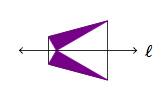# Origami equilateral triangle

Alignments to Content Standards: G-CO.D.13 G-CO.D.12

Jessica is working to construct an equilateral triangle with origami paper and uses the following steps.

1. First she folds the paper in half and then unfolds it , leaving the crease along the dotted line:2. Next Jessica folds two corners over to the center crease as shown in the picture below:Explain why the two purple triangles meet in a point as the picture suggests and why the large white triangle is an equilateral triangle.

## IM Commentary

The purpose of this task is to explore reflections in the context of paper folding. Each fold is associated with a line of reflection and understanding each step in the construction requires careful analysis of angles and of the properties of reflections.

Origami is a great source of problems for developing geometric intuition, cultivating artistic skills, and also for careful mathematical reasoning about reflections. Although the main alignment for this task is G-CO.12, all work with origami also relates to G-CO.A, ''Experiment with Transformations of the Plane,'' and G-SRT.5, ''Use congruence and similarity criteria for triangles to solve problems and to prove relationships in geometric figures.'' Although students do not need origami paper in order to work on this task, it would definitely be beneficial to have some paper squares with which to experiment. A variety of approaches are possible, with two presented in the solutions and a separate method is discussed below in the commentary. Work like this with origami can be done either before or after formal work with geometric proofs: if done before, it can serve as motivation and afterward it provides a rich source of examples for proving geometric theorems.

Note that often in origami the goal is not only to create a shape, like an equilateral triangle, but also to fold the paper up completely into the desired shape. Jessica has not achieved this additional goal but a slight adaptation of her construction does. Below is a picture of steps to fold the paper square into an equilateral triangle shape:Picture (a) shows folding the square in half in both directions, leaving two creases. At the next step, shown in picture (b), two sides of the square are folded inward to meet the creases from step (a). Picture (c) shows the two creases left in step (b) and the new crease needed to fold the square into an equilateral triangle.

The Standards for Mathematical Practice focus on the nature of the learning experiences by attending to the thinking processes and habits of mind that students need to develop in order to attain a deep and flexible understanding of mathematics. Certain tasks lend themselves to the demonstration of specific practices by students. The practices that are observable during exploration of a task depend on how instruction unfolds in the classroom. While it is possible that tasks may be connected to several practices, the commentary will spotlight one practice connection in depth. Possible secondary practice connections may be discussed but not in the same degree of detail.

Students engage in Mathematical Practice Standard 5, Use appropriate  tools strategically, by considering a tool’s usefulness, its strengths and limitations, as well as knowing how to use it appropriately. This task examines the mathematics of the paper folding of a square piece of paper resulting in the creation of an equilateral triangle. Folding paper allows students to analyze the resulting shapes by focusing on angles and the properties of reflection. This experimentation with paper folding allows students to explore and discuss observations before formulating an argument about Jessica’s triangle. Teachers may guide this exploration with questions such as: “What do we know about squares and equilateral triangles?” and “How might those attributes help us explain why the two purple triangles meet in a point as the picture suggests and why the large white triangle is an equilateral triangle?”

As students investigate this task through paper folding, they will ''Look for and express regularity in repeated reasoning'' (MP8) as the folds they make need to repeated twice in order to produce the equilateral triangle and thus their reasoning about one side of the triangle can be repeated for the other side. Students will also ''Reason abstractly and quantitatively'' (MP2) if they choose to represent the paper folding geometrically in order to calculate angles as seen in Solution 2.

## Solutions

Solution: 1 Studying Side Lengths

In the picture below the crease for the first fold is extended to a line labelled $\ell$:Since $\ell$ connects midpoints of opposite sides of the square reflection about $\ell$ is a symmetry for the square. Reflection about $\ell$ switches the two purple triangles and this means that they must meet at a single point on $\ell$ as the picture suggests.

To see why the white triangle is equilateral, we label its vertices as in the picture below:Note that $|PQ| = |QR| = |PR|$ as all three segments are sides of our original shape which was assumed to be a square. So $\triangle PQR$ is equilateral.

Solution: 2 Measuring Angles

We begin by labelling several important points on the (un)folded square.Here $B$ is the point where the two purple triangles meet and $\overline{BC}$ meets the side of the square in a right angle. For $\triangle ABC$ we have $|AB| = s$, the side length of the square, and $|BC| = \frac{s}{2}$ since the fold line is a reflective symmetry of the square. Hence $$\sin{\angle CAB} = \frac{1}{2}$$ so $\angle CAB$ is a 30 degree angle. We know that $m(\angle DAC) = 90$ and so $m(\angle DAB) = 60$. We can also show that $m(\angle ADB) = 60$: this can be done by repeating the argument for $\angle DAB$ or by using the horizontal crease in the square as reflection about this line interchanges angles $DAB$ and $ADB$. Since the sum of the angles of $\triangle ADB$ is 180 degrees, $m(\angle DBA) = 60$ as well and $\triangle ADB$ is an equilateral triangle.